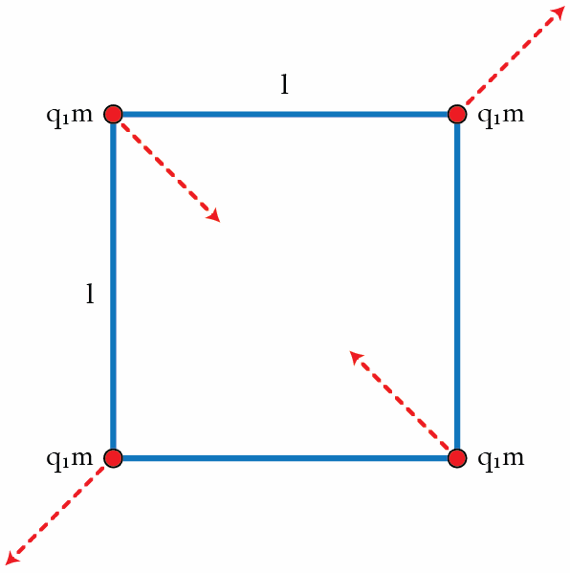# Oscillations of a tetra-atomic molecule

Consider the following model of a tetra-atomic molecule: Four point-charges with charge $q$ and mass $m$ are connected by light rigid rods of length $l$. Clearly, the molecule is in equilibrium when the charges form a square of side $l$. Since this equilibrium is stable, the molecule can oscillate as shown in the figure. If the parameters $q$, $m$, and $l$ satisfy $\frac{k q^{2}}{m l^{3}}=10^{4}~\mbox{s}^{-2} \quad \textrm{where} \quad k=\frac{1}{4\pi \epsilon_{0}},$ find the period $T$ in seconds of small oscillations.×